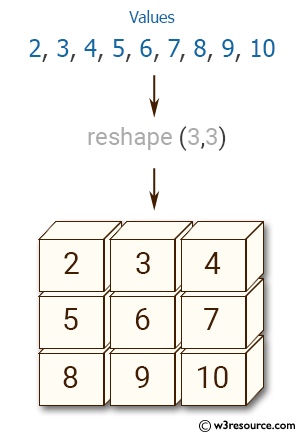﻿ NumPy: Create a 3x3 matrix with values ranging from 2 to 10 - w3resource# NumPy: Create a 3x3 matrix with values ranging from 2 to 10

## NumPy: Array Object Exercise-3 with Solution

Write a NumPy program to create a 3x3 matrix with values ranging from 2 to 10.Sample Solution:-

Python Code:

``````
import numpy as np
x =  np.arange(2, 11).reshape(3,3)
print(x)
```
```

Sample Output:

```[[ 2  3  4]
[ 5  6  7]
[ 8  9 10]]
```

Python Code Editor:

Have another way to solve this solution? Contribute your code (and comments) through Disqus.

What is the difficulty level of this exercise?

Test your Python skills with w3resource's quiz

﻿

## Python: Tips of the Day

Set comprehension:

```>>> m = {x ** 2 for x in range(5)}
>>> m
{0, 1, 4, 9, 16}
```